## Example Questions

### Example Question #193 : Arithmetic

Apples are sold by whole bushels. You cannot purchase part of a bushel. There are 126 apples in a bushel.

Sam is a caterer who needs to bake 300 pies to sell at the county fair. If it takes 4 apples to make a pie, how many bushels must Sam order to ensure he has enough apples for his pies?

9

9.5

10

11

12

10

Explanation:

Because Sam needs to make 300 pies, and each pie needs 4 apples, the number of apples he needs is

300 x 4 = 1200.

To determine how many bushels Sam needs, divide the total number of apples by the number of apples sold in a bushel.

1200 / 126 = 9.524

Because apples are sold by the whole bushel, Sam cannot order part of a bushel. In order to make sure he has sufficient apples, he will need to order 10 bushels.

### Example Question #131 : Integers

Which of the following integers is divisible by?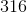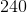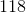Explanation:

In order to find a number divisible by 6, you must find a number divisible by both of its factors — 2 and 3. Only even numbers are divisible by 2, so 81 is eliminated. In order to be divisible by 3, the sum of the digits has to be divisible by 3.

The sum of the digits of 316 is  3 + 1 + 6 = 10.

For 240, the sum is 2 + 4 = 6.

For 118, the sum is 1 + 1 + 8 = 10.

Only 6 is divisible by 3.

### Example Question #198 : Arithmetic

Which of the following rules makes the expression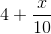an integer?divided byhas a remainder ofis a multiple ofNone of these rules makesan integer.divided byhas a remainder ofAll of these rules makean integer.divided byhas a remainder ofExplanation:

4 is already an integer, so we need to make sure x/10 is an integer too.

Multiples of 5 won't work. For example, 5 is a multiple of 5 but 5/10 isn't an integer. Similarly, if x/10 leaves a remainder of 5, x/10 isn't an integer. For example, 15/10 leaves a remainder of 5 and isn't an integer.

If x/10 has no remainder, then it must be an integer. For example, 10/10 and 20/10 both leave no remainders and simplify to the integers 1 and 2, respectively.

### Example Question #199 : Arithmetic

The remainder of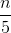is.

Quantity A:Quantity B:Quantity A is larger.

The relationship between the two quantities cannot be determined.

Quantity B is larger.

The two quantities are equal.

The relationship between the two quantities cannot be determined.

Explanation:

If the remainder ofis, we know thatcould be: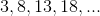Since this generates an entire list of values, we cannot know which quantity is larger.

Do not be tricked by the question, which is trying to get you to say that they are equal!

### Example Question #1 : How To Divide Integers

The remainder of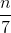is.

The remainder of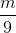is.

Which of the following is a potential value for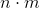?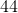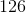Explanation:

Begin by writing out a few possible values forand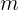.

Since the remainder ofis, we can list: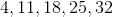Since the remainder ofis, we can list: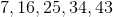Since(which is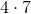) is your smallest possible value, you know thatandare not options.  You cannot derive eitherorfrom the values given.

Therefore, the only option that is left is, which is equal to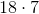.